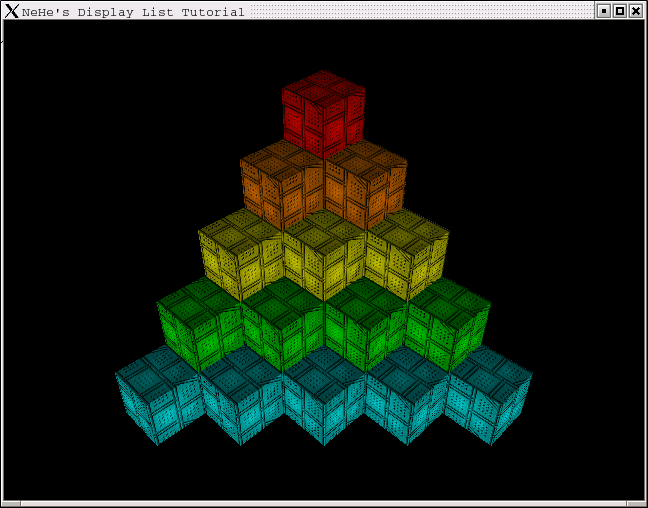## 显示列表### NeHeWidget类

（由nehewidget.h展开。）

```class NeHeWidget : public QGLWidget
{
Q_OBJECT

public:

NeHeWidget( QWidget* parent = 0, const char* name = 0, bool fs = false );
~NeHeWidget();

protected:

void initializeGL();
void paintGL();
void resizeGL( int width, int height );

void keyPressEvent( QKeyEvent *e );
void buildLists();
```

```protected:

bool fullscreen;

GLfloat xRot, yRot, zRot;
GLuint box, top;
```

```  GLuint xLoop, yLoop;
```

```  GLuint texture;
};
```

（由nehewidget.cpp展开。）

```static GLfloat boxcol =
{
{ 1.0, 0.0, 0.0 },
{ 1.0, 0.5, 0.0 },
{ 1.0, 1.0, 0.0 },
{ 0.0, 1.0, 0.0 },
{ 0.0, 1.0, 1.0 }
};

static GLfloat topcol =
{
{ 0.5, 0.0, 0.0 },
{ 0.5, 0.25, 0.0 },
{ 0.5, 0.5, 0.0 },
{ 0.0, 0.5, 0.0 },
{ 0.0, 0.5, 0.5 }
};
```

```NeHeWidget::NeHeWidget( QWidget* parent, const char* name, bool fs )
: QGLWidget( parent, name )
{
xRot = yRot = zRot = 0.0;
box = top = 0;

xLoop = yLoop = 0;

fullscreen = fs;
setGeometry( 0, 0, 640, 480 );
setCaption( "NeHe's Display List Tutorial" );

if ( fullscreen )
showFullScreen();
}
```

```void NeHeWidget::loadGLTextures()
{
QImage tex, buf;
if ( !buf.load( "./data/Cube.bmp" ) )
{
qWarning( "Could not read image file, using single-color instead." );
QImage dummy( 128, 128, 32 );
dummy.fill( Qt::green.rgb() );
buf = dummy;
}
tex = QGLWidget::convertToGLFormat( buf );

glGenTextures( 1, &texture );

glBindTexture( GL_TEXTURE_2D, texture );
glTexParameteri( GL_TEXTURE_2D, GL_TEXTURE_MIN_FILTER, GL_LINEAR );
glTexParameteri( GL_TEXTURE_2D, GL_TEXTURE_MAG_FILTER, GL_LINEAR );
glTexImage2D( GL_TEXTURE_2D, 0, 3, tex.width(), tex.height(), 0,
GL_RGBA, GL_UNSIGNED_BYTE, tex.bits() );
}
```

```void NeHeWidget::buildLists()
{
box = glGenLists( 2 );
```

```  glNewList( box, GL_COMPILE );
```

GL_COMPILE类似于编程。在你写程序的时候，把它装载到编译器里，你每次运行程序都需要重新编译。而如果它已经编译成了.exe文件，那么每次你只需要点击那个.exe文件就可以运行它了，不需要编译。当OpenGL编译过显示列表后，就不需要再每次显示的时候重新编译它了。这就是为什么用显示列表可以加快速度。

```  glBegin( GL_QUADS );
```

```    glNormal3f( 0.0, -1.0, 0.0 );
glTexCoord2f( 1.0, 1.0 ); glVertex3f( -1.0, -1.0, -1.0 );
glTexCoord2f( 0.0, 1.0 ); glVertex3f(  1.0, -1.0, -1.0 );
glTexCoord2f( 0.0, 0.0 ); glVertex3f(  1.0, -1.0,  1.0 );
glTexCoord2f( 1.0, 0.0 ); glVertex3f( -1.0, -1.0,  1.0 );

glNormal3f( 0.0, 0.0, 1.0 );
glTexCoord2f( 0.0, 0.0 ); glVertex3f( -1.0, -1.0,  1.0 );
glTexCoord2f( 1.0, 0.0 ); glVertex3f(  1.0, -1.0,  1.0 );
glTexCoord2f( 1.0, 1.0 ); glVertex3f(  1.0,  1.0,  1.0 );
glTexCoord2f( 0.0, 1.0 ); glVertex3f( -1.0,  1.0,  1.0 );

glNormal3f( 0.0, 0.0, -1.0 );
glTexCoord2f( 1.0, 0.0 ); glVertex3f( -1.0, -1.0, -1.0 );
glTexCoord2f( 1.0, 1.0 ); glVertex3f( -1.0,  1.0, -1.0 );
glTexCoord2f( 0.0, 1.0 ); glVertex3f(  1.0,  1.0, -1.0 );
glTexCoord2f( 0.0, 0.0 ); glVertex3f(  1.0, -1.0, -1.0 );

glNormal3f( 1.0, 0.0, 0.0 );
glTexCoord2f( 1.0, 0.0 ); glVertex3f(  1.0, -1.0, -1.0 );
glTexCoord2f( 1.0, 1.0 ); glVertex3f(  1.0,  1.0, -1.0 );
glTexCoord2f( 0.0, 1.0 ); glVertex3f(  1.0,  1.0,  1.0 );
glTexCoord2f( 0.0, 0.0 ); glVertex3f(  1.0, -1.0,  1.0 );

glNormal3f( -1.0, 0.0, 0.0 );
glTexCoord2f( 0.0, 0.0 ); glVertex3f( -1.0, -1.0, -1.0 );
glTexCoord2f( 1.0, 0.0 ); glVertex3f( -1.0, -1.0,  1.0 );
glTexCoord2f( 1.0, 1.0 ); glVertex3f( -1.0,  1.0,  1.0 );
glTexCoord2f( 0.0, 1.0 ); glVertex3f( -1.0,  1.0, -1.0 );
glEnd();

glEndList();
```

```  top = box + 1;
```

```  glNewList( top, GL_COMPILE );

```

```    glNormal3f( 0.0, 1.0, 0.0 );
glTexCoord2f( 0.0, 1.0 ); glVertex3f( -1.0,  1.0, -1.0 );
glTexCoord2f( 0.0, 0.0 ); glVertex3f( -1.0,  1.0,  1.0 );
glTexCoord2f( 1.0, 0.0 ); glVertex3f(  1.0,  1.0,  1.0 );
glTexCoord2f( 1.0, 1.0 ); glVertex3f(  1.0,  1.0, -1.0 );
glEnd();

glEndList();
```

```}
```
```void NeHeWidget::initializeGL()
{
buildLists();
```

```  glEnable( GL_TEXTURE_2D );
glClearColor( 0.0, 0.0, 0.0, 0.5 );
glClearDepth( 1.0 );
glEnable( GL_DEPTH_TEST );
glDepthFunc( GL_LEQUAL );
glEnable( GL_LIGHT0 );
glEnable( GL_LIGHTING );
glEnable( GL_COLOR_MATERIAL );
```

```  glHint( GL_PERSPECTIVE_CORRECTION_HINT, GL_NICEST );
```

```}
```

```void NeHeWidget::paintGL()
{
glClear( GL_COLOR_BUFFER_BIT | GL_DEPTH_BUFFER_BIT );
glBindTexture( GL_TEXTURE_2D, texture );
```

```  for ( yLoop = 1; yLoop < 6; yLoop++ )
{
```

```    for ( xLoop = 0; xLoop < yLoop; xLoop++ )
{
```

```      glLoadIdentity();
glTranslatef( 1.4 + (float(xLoop) * 2.8) - (float(yLoop) * 1.4),
( (6.0 - (float(yLoop)) ) * 2.4 ) - 7.0, -20.0 );
glRotatef( 45.0 - (2.0 * yLoop) + xRot, 1.0, 0.0, 0.0 );
glRotatef( 45.0 + yRot, 0.0, 1.0, 0.0 );
```

```      glColor3fv( boxcol[yLoop-1] );
```

```      glCallList( box );
```

```      glColor3fv( topcol[yLoop-1] );
glCallList( top );
}
}
}
```
```void NeHeWidget::keyPressEvent( QKeyEvent *e )
{
switch ( e->key() )
{
case Qt::Key_Up:
xRot -= 0.2;
updateGL();
break;
case Qt::Key_Down:
xRot += 0.2;
updateGL();
break;
case Qt::Key_Left:
yRot -= 0.2;
updateGL();
break;
case Qt::Key_Right:
yRot += 0.2;
updateGL();
break;
case Qt::Key_F2:
fullscreen = !fullscreen;
if ( fullscreen )
{
showFullScreen();
}
else
{
showNormal();
setGeometry( 0, 0, 640, 480 );
}
update();
break;
case Qt::Key_Escape:
close();
}
}
```